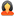Question

All Subjects Statistics General Statistics WEB CALCULATOR EXERCISE 2

Offered Price \$ 40.00

WEB CALCULATOR EXERCISE 2

Question # 00001022
Subject: Statistics
Topic: General Statistics
Due on: 09/14/2013
Posted On: 09/12/2013 08:21 PM

Rating:
4.1/5Posted ByQuestions:
6
Tutorials:
7
Feedback Score:

Question

Web Calculator Exercise 2

Z test & One sample t-test

1. A researcher is interested in whether students who attend private high schools have higher average SAT Scores than students in the general population. A random sample of 90 students at a private high school is tested and and a mean SAT score of 1030 is obtained. The average score for public high school student is 1000 (?= 200).

a. Is this a one- or two tailed test?

b. What are H0 and Ha for this study?

c. Compute Z obt

d. What is the Z critical value (Z cv ) using a 0.05 alpha level?

e. Should H0 be rejected? What should the researcher conclude?

f. Determine the 95 % confidence interval for the population mean, based on the sample mean.

2. A researcher hypothesized that the pulse rates of long-distance athletes differ from those of other athletes. He believed that the runners’ pulses would be slower. He obtained a random sample of 10 long-distance runners. He measured their resting pulses. Their pulses were 45, 45, 64, 50, 58, 49, 47, 55, 50, 52 beats per minute. The average resting pulse of athletes in the general population is normally distributed with a pulse rate of 60 beats per minute.

a. What statistical test should be used to analyze the data?

b. Is this a one- or two- tailed test?

c. What are H0and Ha for this study?

d. Find tcv from appendix A in Jackson’s text.

e. Compute t obt

f. Should H0 be rejected? What should the researcher conclude?

3. A researcher hypothesizes that people who listen to music via headphones have greater hearing loss and will thus score lower on a hearing test than those in the general population. On a standard hearing test, the overall mean for the general population is 22.5. The researcher gives this same test to a random sample of 12 individuals who regularly use headphones. Their scores on the test are 15, 14, 20, 20, 25, 22, 21, 19, 16, 17, 21, 22.

a. What statistical test should be used to analyze the data?

b. Is this a one- or two- tailed test?

c. What are H0and Ha for this study?

d. Compute t obt

e. Should H0 be rejected? What should the researcher conclude?

Tutorials for this Question
Available for
\$ 40.00

WEB CALCULATOR EXERCISE 2

Tutorial # 00000885
Posted On: 09/12/2013 08:22 PM
Posted By:StatisticsAcerQuestions:
6
Tutorials:
7
Feedback Score:
Tutorial Preview … a…
Attachments
Web_Calculator_Exercise_2-20130225-222025.doc (76 KB)
Preview: (988.68, 1071.32) A researcher hypothesized that the .....
* - Additional Paypal / Transaction Handling Fee (3.9% of Tutorial price + \$0.30) applicable